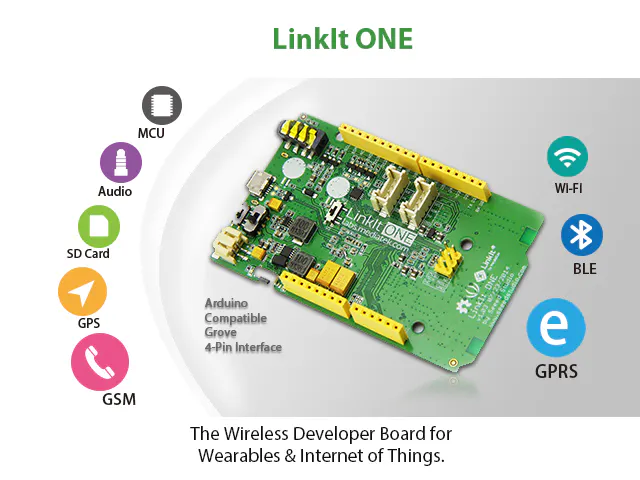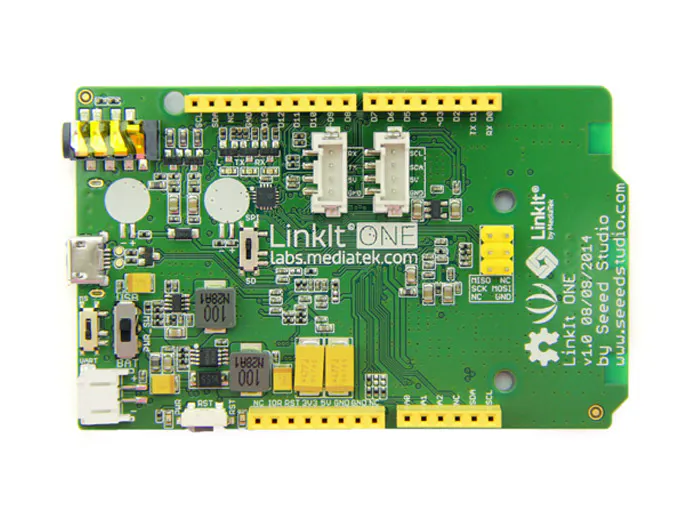Online GPS datalogger with Linkit ONE

Take continusly your postion and store it on a remote server

EasyProtip1,473Things used in this project

Hardware components×1

Code

C/C++
```#include <LGPS.h>
#include <LGPRS.h>
#include <LGPRSClient.h>

gpsSentenceInfoStruct info; //needed to get GPS data

double latitude = 0.00;
double longitude = 0.00;
float altitude = 0.00;
float dop = 100.00; //dilution of precision
float geoid = 0.00;
float k_speed = 0.00, m_speed = 0.00; //speed in knots and speed in m/s
float track_angle = 0.00;
int fix = 0;
int hour = 0, minute = 0, second = 0;
int sat_num = 0; //number of visible satellites
int day = 0, month = 0, year = 0;
String time_format = "00:00:00", date_format = "00:00:0000";
String lat_format = "0.00000", lon_format = "0.00000";
char file = "";
int pause = 3000; //time in milliseconds between two logs

char server[] = "yourserver.com";
int port = 80; // HTTP
LGPRSClient client;

void setup()
{
Serial.begin(115200);
LGPS.powerOn();
Serial.println("GPS started.");
Serial.print("Attaching GPRS...");
while (!LGPRS.attachGPRS())delay(500);
Serial.println("OK.");
}
void loop()
{
if (getData(&info) > 3)
{
if (client.connect(server, port))
{
Serial.println("connected");
String str = "GET /store_data.php?";
str += "date=";
str += date_format;
str += "&time=";
str += time_format;
str += "&lat=";
str += lat_format;
str += "&lon=";
str += lon_format;
client.print(str);
client.println(" HTTP/1.1");
client.print("Host: ");
client.println(server);
client.println("Connection: close");
client.println();
}
else Serial.println("Unable to connect");
}
else Serial.println("Less then 4 satelites.");
delay(pause);
}
/**
*Converts degrees from (d)ddmm.mmmm to (d)dd.mmmmmm
*@param str the string rappresentation of the angle in (d)ddmm.mmmm format
*@param dir if true the direction is south, and the angle is negative.
*@return the given angle in dd.mmmmmm format.
*/
float convert(String str, boolean dir)
{
double mm, dd;
int point = str.indexOf('.');
dd = str.substring(0, (point - 2)).toFloat();
mm = str.substring(point - 2).toFloat() / 60.00;
return (dir ? -1 : 1) * (dd + mm);
}
/**
*Gets gps informations
*@param info gpsSentenceInfoStruct is a struct containing NMEA sentence infomation
*@return the number of hooked satellites, or 0 if there was an error getting informations
*/
int getData(gpsSentenceInfoStruct* info)
{
Serial.println("Collecting GPS data.");
LGPS.getData(info);
Serial.println((char*)info->GPGGA);
if (info->GPGGA == '\$')
{
Serial.print("Parsing GGA data....");
String str = (char*)(info->GPGGA);
str = str.substring(str.indexOf(',') + 1);
hour = str.substring(0, 2).toInt();
minute = str.substring(2, 4).toInt();
second = str.substring(4, 6).toInt();
time_format = "";
time_format += hour;
time_format += ":";
time_format += minute;
time_format += ":";
time_format += second;
str = str.substring(str.indexOf(',') + 1);
latitude = convert(str.substring(0, str.indexOf(',')), str.charAt(str.indexOf(',') + 1) == 'S');
int val = latitude * 1000000;
String s = String(val);
lat_format = s.substring(0, (abs(latitude) < 100) ? 2 : 3);
lat_format += '.';
lat_format += s.substring((abs(latitude) < 100) ? 2 : 3);
str = str.substring(str.indexOf(',') + 3);
longitude = convert(str.substring(0, str.indexOf(',')), str.charAt(str.indexOf(',') + 1) == 'W');
val = longitude * 1000000;
s = String(val);
lon_format = s.substring(0, (abs(longitude) < 100) ? 2 : 3);
lon_format += '.';
lon_format += s.substring((abs(longitude) < 100) ? 2 : 3);
str = str.substring(str.indexOf(',') + 3);
fix = str.charAt(0) - 48;
str = str.substring(2);
sat_num = str.substring(0, 2).toInt();
str = str.substring(3);
dop = str.substring(0, str.indexOf(',')).toFloat();
str = str.substring(str.indexOf(',') + 1);
altitude = str.substring(0, str.indexOf(',')).toFloat();
str = str.substring(str.indexOf(',') + 3);
geoid = str.substring(0, str.indexOf(',')).toFloat();
Serial.println("done.");
if (info->GPRMC == '\$')
{
Serial.print("Parsing RMC data....");
str = (char*)(info->GPRMC);
int comma = 0;
for (int i = 0; i < 60; ++i)
{
if (info->GPRMC[i] == ',')
{
comma++;
if (comma == 7)
{
comma = i + 1;
break;
}
}
}
str = str.substring(comma);
k_speed = str.substring(0, str.indexOf(',')).toFloat();
m_speed = k_speed * 0.514;
str = str.substring(str.indexOf(',') + 1);
track_angle = str.substring(0, str.indexOf(',')).toFloat();
str = str.substring(str.indexOf(',') + 1);
day = str.substring(0, 2).toInt();
month = str.substring(2, 4).toInt();
year = str.substring(4, 6).toInt();
date_format = "20";
date_format += year;
date_format += "-";
date_format += month;
date_format += "-";
date_format += day;
Serial.println("done.");
return sat_num;
}
}
else
{
Serial.println("No GGA data");
}
return 0;
}
```

store_data.php

PHP
This is the php file on your server, which will process requests from linkit ONE
```<?php

function getParameter(\$par, \$default = null)
{
if (isset(\$_POST[\$par]) && strlen(\$_POST[\$par])) return \$_POST[\$par];
if (isset(\$_GET[\$par]) && strlen(\$_GET[\$par])) return \$_GET[\$par];
else return \$default;
}

\$param_names = array("date", "time","lat","lon");
\$file = getParameter("date").".txt";
\$line="";
for(\$i=0; \$i< count(\$param_names); \$i++)
{
\$line = \$line.getParameter(\$param_names[\$i]);
if(\$i<count(\$param_names)-1)\$line = \$line.",";
}
\$line = \$line."\n";
file_put_contents(\$file, \$line, FILE_APPEND | LOCK_EX);

?>
```